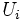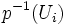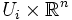Vector bundle

A vector bundle over a topological space$B$ is a topological space$E$ with a bundle map$p:E \to B$, along with an open cover$U_i$ of$B$ such that:
1. For every$i$, the projection map from$p^{-1}(U_i)$ to$U_i$ looks like a coordinate projection from$U_i \times \R^n$ to$U_i$